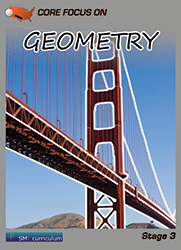#### Geometry

In this course, students learn that there are numbers that are not rational, and approximate them by rational numbers.  They work with radicals and integer exponents and use this knowledge to understand and apply the Pythagorean Theorem.  Students use transformations to show congruence and similarity.   At the end of the course, students solve real-world and mathematical problems involving volume of cylinders, cones and spheres.

### Textbook & Stage I Series Pricing

1 FREE Teacher Edition(TE) and Teacher Resource Binder(TR) per 40 student texts ordered!